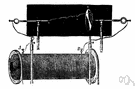# inductance

(redirected from Electric mutual induction)
Also found in: Thesaurus, Medical, Encyclopedia.

## in·duc·tance

(ĭn-dŭk′təns)
n.
1. The property of an electric circuit by which an electromotive force is induced in it as the result of a changing magnetic flux.
2. A circuit element, typically a conducting coil, in which electromotive force is generated by electromagnetic induction.

## inductance

(ɪnˈdʌktəns)
n
1. (General Physics) Also called: induction the property of an electric circuit as a result of which an electromotive force is created by a change of current in the same circuit (self-inductance) or in a neighbouring circuit (mutual inductance). It is usually measured in henries. Symbol: L See also self-inductance, mutual inductance
2. (General Physics) another name for inductor

## in•duct•ance

(ɪnˈdʌk təns)

n.
1. the property of a circuit by which a change in current induces, by electromagnetic induction, an electromotive force.
[1885–90]

## in·duc·tance

(ĭn-dŭk′təns)
The property of an electric circuit that makes it possible for an electromotive force to be created in a nearby circuit by a change of current in either circuit.
ThesaurusAntonymsRelated WordsSynonymsLegend:
 Noun 1 inductance - an electrical phenomenon whereby an electromotive force (EMF) is generated in a closed circuit by a change in the flow of currentinductionelectrical phenomenon - a physical phenomenon involving electricitymutual induction - generation of electromotive forces in each other by two adjacent circuitsself-induction - generation of an electromotive force (EMF) in a circuit by changing the current in that circuit; usually measured in henries 2inductance - an electrical device (typically a conducting coil) that introduces inductance into a circuitinductorelectrical device - a device that produces or is powered by electricity
Translations
Site: Follow: Share:
Open / Close